# GED Math : Patterns and Sequences

## Example Questions

← Previous 1 3

### Example Question #1 : Patterns And Sequences

What is the next number in the series?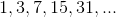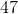39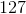Explanation:

To pattern uses the formula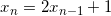.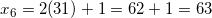### Example Question #2 : Patterns And Sequences

Find the next number in the sequence: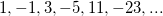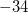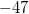Explanation:

The pattern of the sequence is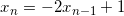.

Therefore,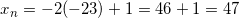.

### Example Question #3 : Patterns And Sequences

What is the next number in the sequence?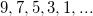Explanation:

The pattern follows this equation: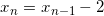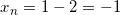### Example Question #1 : Patterns And Sequences

What property of arithmetic is symbolized below?

If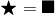, then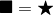Reflexive

Associative

Commutative

Symmetric

Symmetric

Explanation:

The symbols show that if one number is equal to another,  the equality holds no matter the order of the two. This is the symmetric property of equality.

### Example Question #1 : Patterns And Sequences

What property of arithmetic is symbolized below?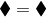Identity

Transitive

Commutative

Reflexive

Reflexive

Explanation:

The symbols express the idea that any number is equal to itself. This is the reflexive property of equality.

### Example Question #1 : Patterns And Sequences

What property of arithmetic is symbolized below?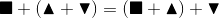Transitive

Associative

Commutative

Symmetric

Associative

Explanation:

The symbols express the idea that if three numbers are added, the same sum results regardless of which numbers are added first. This is the associative property of addition.

### Example Question #7 : Patterns And Sequences

What number replaces the circle in the following sequence?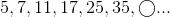Explanation:

Add 2 to the first number to get the second; each subsequent increment is increased by 2. The pattern: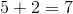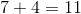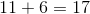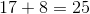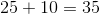Add 12 to get the next element: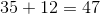, which is the correct choice.

### Example Question #8 : Patterns And Sequences

What number replaces the circle in this sequence?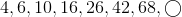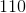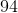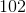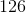Explanation:

After the first two elements, each subsequent element is the sum of the previous two (similar to the Fibonacci sequence).

The third and subsequent elements are calculated as follows: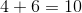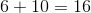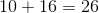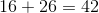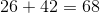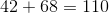, the correct response.

### Example Question #1 : Patterns And Sequences

What number replaces the circle in the following sequence?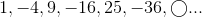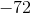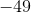Explanation:

The sequence consists of the perfect square integer, except that every other element is negated. The sequence goes like this: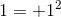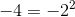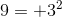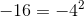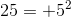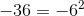The next entry is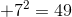.

### Example Question #10 : Patterns And Sequences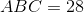, andare distinct integers.

Which of the following could be equal to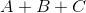?Explanation:

We need to find ways to factor 28 such that the three factors are different, and then find the sum of those factors in each case.

28 can be expressed as the product of three integers four ways:

I)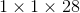II)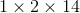III)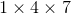IV)We disregard (I) and (IV) since the factors are not distinct.

We look at (II) and (III):

II: Sum: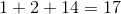III: Sum: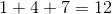Of the four choices, only 17 is a possible sum; that is the correct choice.

← Previous 1 3

### All GED Math Resources